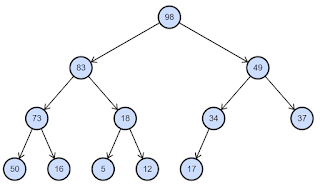## Saturday, 17 October 2020

### How To Solve "Caused by: org.hibernate.HibernateException: Missing table" When Table Is Present In Database

If you are using JPA or Hibernate directly and got that exception while starting your application, there is one obvious reason for that. You forgot to add that table in MySQL database or any other database that you are using. But if the table is present, then most likely it is a database user permission issue. Suppose you are using MySQL, then you will have to provide a MySQL username & password with database connection url so that Hibernate can connect to the database from your Java application. That might be different than the MySQL user you used to connect to the database directly & create the table. To check, use the same MySQL username & password used in your Java application & connect to database console directly. Run a select query on the table. If you get some access denied error like "ERROR 1142 (42000): SELECT command denied to user", that means you need to grant access to this database user for this particular table. And that should solve the problem.

## Thursday, 15 October 2020

### Building Binary Heap In O(n) Worst Time Complexity Explained

There are two ways we can build a binary heap, top-down & bottom-up. We will take top-down approach first. Let's consider the max heap below.For top-down approach we will start from the root & then move the node towards the bottom till max heap property is satisfied. As you can see from screenshot, heap is a complete binary tree.
We will denote number of nodes at level h as n(h).
When h = 0, n(h) = 2^0 = 1
h = 1, n(h) = 2^1 = 2
h = 2, n(h) = 2^2 = 4
As you can see, a particular level always contains one more node than the total nodes of all previous levels. Let's consider when height is 2. n(h) is 4 which is greater than (1 + 2) = 3 which is total number of nodes in height 0 & 1.
But because heap is a complete binary tree, last level might not have all the nodes as you can see in the screenshot.
When h = 3, n(h) = 5
But what about total number of leaf nodes? At h=3, we have 5 leaf nodes & h=2 we have 1 leaf node.
So total leaf node is 6. And total non-leaf nodes is also 6. If last level contains all possible nodes, then more than half of total nodes are leaf nodes. Otherwise, we will have at least half nodes as leaf nodes in a complete binary tree. This is an important thing to understand.

Now let's go back to our top down approach. We start from the root each time. And then move nodes towards leaf level till it satisfies heap property. So we will have to move at least half of the nodes to leaf level from root level which is basically height of the tree h & that is log(n). So this scenario will take (n / 2) * log(n) time. So time complexity will become O(n log(n)).

We will take a look at bottom-up approach now. For bottom-up, we will start at the leaf level. At leaf level, we don't need to do any shift as any leaf node would satisfy heap property. We move one level up (h-1) & at that level we need to shift at most one level down. At (h-2) level we need to shift at most 2 level.
So we can write something similar as below:
(0 * n/2) + (1 * n/4) + (2 * n/8) + (3 * n/16) + ... + (h * 1)
= (1 * n/4) + (2 * n/8) +  (3 * n/16) + ... + (h * 1)
h
= ∑ kn/(2^(k+1))
k=1
We can move n out
h
n * ∑ k/(2^(k+1))
k=1

k/(2^(k+1)) can be simplified as k/(2^k * 2). 2 can be omitted as we don't need to consider constant value for time complexity.

h
∑ k/(2^k)) we need to check what happens when k value approaches to infinity.
k=1

1/ 2^1 + 2/2^2 + 3/2^3 + 4/2^4 + 5/^2^5 + 6/2^6 + ....
=1/2 + 1/2 + 3/8 + 1/4 + 5/32 + 3/32 + ....

As you can see the value will converge towards 2.
So worst time complexity will become O(n * 2) which is basically O(n).

## Wednesday, 7 October 2020

### What Is The Actual Difference Between Closeable And AutoCloseable In Java

Well, practically there is not much difference. If you check Java doc, you will notice that Closeable interface extends AutoCloseable interface. But there is one difference & that explains the reason why AutoCloseable interface was introduced in Java 7. Closeable  close() method can throw IOException only. And that made  sense as you will be using Closeable mostly with IO resources. But to handle other generic exceptions too with close() method, AutoCloseable was introduced.

public interface Closeable extends AutoCloseable {

public void close() throws IOException;

}

public interface AutoCloseable {

void close() throws Exception;

}

There is a misconception that Closeable can't be used with try-with-resources code block. But that is not true from Java 7 & hopefully everybody has a more upgraded version than that 😀. Both interfaces work with try-with-resources statement so that you don't have to call close() method explicitly.

### Java Program To Solve Maximum Size Rectangle In Binary Matrix

Given a binary matrix, find max rectangle with all 1's. A : [ 1 1 1 0 0 1 1 0 1 1 1 0 ] Output : 6 Dynamic programmi...